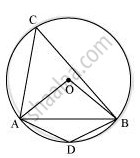# A chord of a circle is equal to the radius of the circle. Find the angle subtended by the chord at a point on the minor arc and also at a point on the major arc. - Mathematics

A chord of a circle is equal to the radius of the circle. Find the angle subtended by the chord at a point on the minor arc and also at a point on the major arc.

#### SolutionIn ΔOAB,

AB = OA = OB = radius

∴ ΔOAB is an equilateral triangle.

Therefore, each interior angle of this triangle will be of 60°.

∴ ∠AOB = 60°

angleACB=1/2angleAOB=1/2(60^@)=30^@

⇒ ∠ADB = 180° − 30° = 150°

Therefore, angle subtended by this chord at a point on the major arc and the minor arc are 30° and 150° respectively.

Is there an error in this question or solution?
Chapter 10: Circles - Exercise 10.5 [Page 185]

#### APPEARS IN

NCERT Class 9 Maths
Chapter 10 Circles
Exercise 10.5 | Q 2 | Page 185

Share